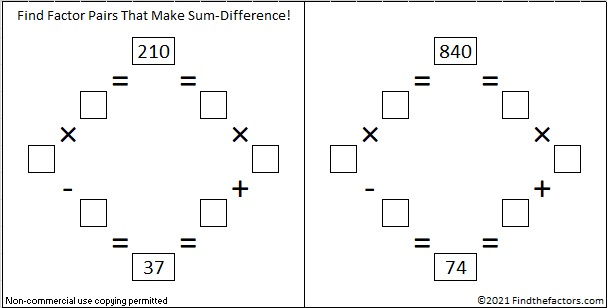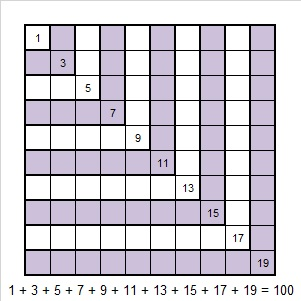# 840 is the Smallest Number that Is the Top Number in THREE Sum-Difference Puzzles

Contents

### Factor Trees for 840:

840 has more factors than any previous number. Those factors will help us write 840 as the sum of consecutive counting numbers, consecutive even numbers, and consecutive odd numbers. What are the factors of 840? Here are a couple of the many possible factor trees for 840:The red leaves on the tree are prime numbers. Gathering the six red leaves from either factor tree above gives us 840’s prime factorization: 840 = 2³ × 3 × 5 × 7.  Now 840 is not the smallest number to have six red leaves. In fact, there are smaller numbers with as many as nine leaves, but 840’s six innocent-looking red leaves will turn into a whopping 32 factors!

### Factors of 840:

• 840 is a composite number.
• Prime factorization: 840 = 2 × 2 × 2 × 3 × 5 × 7, which can be written 840 = 2³ × 3 × 5 × 7.
• The exponents in the prime factorization are 3, 1, 1, and 1. Adding one to each and multiplying we get (3 + 1)(1 + 1)(1 + 1) )(1 + 1) = 4 × 2 × 2 × 2 = 32. Therefore 840 has exactly 32 factors.
• Factors of 840: 1, 2, 3, 4, 5, 6, 7, 8, 10, 12, 14, 15, 20, 21, 24, 28, 30, 35, 40, 42, 56, 60, 70, 84, 105, 120, 140, 168, 210, 280, 420, 840
• Factor pairs: 840 = 1 × 840, 2 × 420, 3 × 280, 4 × 210, 5 × 168, 6 × 140, 7 × 120, 8 × 105, 10 × 84, 12 × 70, 14 × 60, 15 × 56, 20 × 42, 21 × 40, 24 × 35 or 28 × 30
• Taking the factor pair with the largest square number factor, we get √840 = (√4)(√210) = 2√210 ≈ 28.98275.840 is also the smallest number that can be evenly divided by the first eight counting numbers!

### Sum-Difference Puzzles:

210 has eight factor pairs. One of those factor pairs adds up to 29, and another one subtracts to 29. Another factor pair adds up to 37, and a different one subtracts to 37. Thus, 210 produces two different Sum-Difference Puzzles shown in the first two graphics below!

840 is a multiple of 210 and  has sixteen factor pairs! One of the factor pairs adds up to ­58 and another to 74. A different factor pair subtracts to 58, and still a different one subtracts to 74. If you can identify those factor pairs, then you can solve the two puzzles that are next to the 210 puzzles below!

###840 has yet another factor pair that adds up to 113 and a different one that subtracts to 113. If you can find those factor pairs, then you can solve this next primitive puzzle:If you need help with any of those 840 Sum-Difference puzzles, the chart below shows the sums and differences of all of 840’s factor pairs. See which sums also appear in the difference column.### How to find consecutive counting numbers that add up to 840:

840 is more than the 40th triangular number (820) and less than the 41st triangular number (861). We can also arrive at the number 40 by using √(1 + 840×2) – 1 = 40, no rounding necessary. 840 has six odd factors that are not more than 40, namely 1, 3, 5, 7, 15, 21 and 35.

I’ll describe the ways we can write 840 as the sum of consecutive numbers. Can you write out the sums? I’ve done one of them for you:

• using 3 numbers with 280 as the middle number,
• using 5 numbers with 168 as the middle number,
• using 7 numbers with 120 as the middle number; 117 + 118 + 119 + 120 + 121 + 122 + 123 = 840
• using 15 numbers with 56 as the middle number,
• using 21 numbers with 40 as the middle number
• using 35 numbers with 24 as the middle number.

Notice each of those ways has a factor pair of 840 in the description.

The largest power of 2 that is a factor of 840 is 8, which doubled becomes 16. Which of 840’s odd factors multiplied by 16 are not more than 40? 1 × 16 = 16, and 3 × 16 = 48. Oops, that’s too much. The rest of its odd factors times 16 will be too much as well. Nevertheless, we can write 840 as the sum of 16 counting numbers. 840÷16 = 52.5 so 52 and 53 will be the 8th and 9th numbers in the sum.

• 45 + 46 + 47 + 48 + 49 + 50 + 51 + 52 + 53 + 54 + 55 + 56 + 57 + 58 + 59 + 60 = 840

### How do we find consecutive EVEN numbers that add up to an even number?

Only even numbers can be the sum of consecutive even numbers. Let’s use 840 as an example again. 840÷2 = 420.First, we will find all the ways to write 420 as the sum of consecutive numbers. Then we will simply double the middle number and surround it with the appropriate number of even numbers to get a sum of even numbers that add up to 840:

√(1 + 420×2) – 1 = 28, no rounding necessary, so we will make a list of the odd factors that are not more than 28. They are 1, 3, 5, 7, 15, and 21. We also note that the largest power of 2 that is a factor of 420 is 4. Doubling 4, we get 8. Which of 420’s odd factors multiplied by 8 are not more than 28? 1 × 8 = 8, and 3 × 8 = 24. All of the rest will be too much.

I’ll describe the ways we can write 840 as the sum of consecutive even numbers. You can see 420’s factor pairs and 840’s factor pairs in the descriptions. Can you write out the sums? I’ve done one of them for you:

• using 3 even numbers with 2 × 140 = 280 as the middle number
• using 5 even numbers with 2 × 84 = 168 as the middle number
• using 7 even numbers with 2 × 60 = 120 as the middle number
• using 15 even numbers with 2 × 28 = 56 as the middle number
• using 21 even numbers with 2 × 20 = 40 as the middle number; 20 + 22 + 24 + 26 + 28 + 30 + 32 + 34 + 36 + 38 + 40 + 42 + 44 + 46 + 48 + 50 + 52 + 54 + 56 + 58 + 60 = 840

We can also write 840 as the sum of an even amount of consecutive even numbers.

• using 8 even numbers: 2 times (49 + 50 + 51 + 52 + 53 + 54 + 55 + 56 = 420) becomes 98 + 100 + 102 + 104 + 106 + 108 + 110 + 112 = 840. Notice that 840÷8 = 105, the odd number that is between the two numbers in the exact middle of the sum.
• Likewise, using 24 even numbers: 12 + 14 + 16 + 18 + 20 + 22 + 24 + 26 + 28 + 30 + 32 + 34 + 36 + 38 + 40 + 42 + 44 + 46 + 48 + 50 + 52 + 54 + 56 + 58 = 840. Notice that 840÷ 24 = 35, the odd number that is between the two numbers in the exact middle of the sum.

### How to find consecutive ODD numbers that add up to an even number like 841:

Let me first point out that every square number, n², is the sum of the first n numbers.

For example: 100 = 10², and 100 is also the sum of the first 10 odd numbers as illustrated below:A similar statement is true for every other square number:Yes, every square number, n², is the sum of the first n odd numbers.

To write a non-square even number as the sum of consecutive odd numbers, the first thing we must do is determine if the number can be written as the difference of two squares. If an even number has a factor pair, b × a, in which b > a, and BOTH a and b are even, then that even number can be expressed as the difference of two squares by using [(b + a)/2]² – [(b – a)/2]².

Now as long as an even number can be expressed as the difference of two squares, , then that number can be written as the sum of all the odd numbers from 2A+1 to 2B-1.

840 is an even number with eight factor pairs in which both numbers are even. Let’s use those even factor pairs to find the ways to write 840 as the sum of consecutive ODD numbers:

• 420 × 2 = 840 means 211² 209² = 840, and that means the sum of the 2 consecutive odd numbers from 419 to 421 = 840
• 210 × 4 = 840 means 107² 103² = 840, and that means the sum of the 4 consecutive odd numbers from 207 to 213 = 840
• 140 × 6 = 840 means 73² 67² = 840, and that means the sum of the 6 consecutive odd numbers from 135 to 145 = 840
• 84 × 10 = 840 means 47² 37² = 840, and that means the sum of the 10 consecutive odd numbers from 75 to 93 = 840
• 70 × 12 = 840 means 41² 29² = 840, and that means the sum of the 12 consecutive odd numbers from 59 to 81 = 840
• 60 × 14 = 840 means 37² 23² = 840, and that means the sum of the 14 consecutive odd numbers from 47 to 73 = 840
• 42 × 20 = 840 means 31² 11² = 840, and that means the sum of the 20 consecutive odd numbers from 23 to 61 = 840
• 30 × 28 = 840 means 29² = 840, and that means the sum of the 28 consecutive odd numbers from 3 to 29 = 840

Thus, 840 with its record setting 32 factors, can be written as the sum of 7 consecutive numbers, 7 consecutive even numbers, and 8 consecutive odd numbers!

### More about the Number 840:

Incidentally, being able to write 840 as the difference of two squares, eight different ways also makes 840 a leg in at least eight different Pythagorean triples. Those Pythagorean triples can be calculated using the numbers from the difference of two squares. For example, 682-840-1082 can be calculated from 2(31)(11), 31² 11², 31² + 11².

840 was the leg for those eight triples. It is possible that looking at 2(b)(a), where b × a = 420, will produce some more Pythagorean triples with 840 as the leg.

840 is also the hypotenuse of one Pythagorean triple, 504-672-840.

On a note totally unrelated to anything I’ve written above, 840 is a repdigit in two bases:

• SS BASE 29 (S is 28 base 10) Note that 28(29) + 28(1) = 28(30) = 840
• 00 BASE 34 (0 is 24 base 10) Note that 24(34) + 24(1) = 24(35) = 840

840 is also the sum of twin prime numbers 419 and 421.

This site uses Akismet to reduce spam. Learn how your comment data is processed.